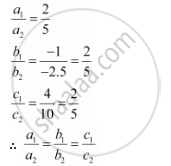Share

# The Planes: 2x − Y + 4z = 5 and 5x − 2.5y + 10z = 6 Are - CBSE (Commerce) Class 12 - Mathematics

ConceptPlane Equation of a Plane in Normal Form

#### Question

The planes: 2− y + 4z = 5 and 5x − 2.5y + 10z = 6 are

(A) Perpendicular

(B) Parallel

(C) intersect y-axis

(C) passes through (0,0,5/4)

#### Solution

The equations of the planes are

2− y + 4z = 5 … (1)

5x − 2.5y + 10z = 6 … (2)

It can be seen that,Therefore, the given planes are parallel.

Hence, the correct answer is B.

Is there an error in this question or solution?

#### APPEARS IN

NCERT Solution for Mathematics Textbook for Class 12 (2018 to Current)
Chapter 11: Three Dimensional Geometry
Q: 23 | Page no. 499
Solution The Planes: 2x − Y + 4z = 5 and 5x − 2.5y + 10z = 6 Are Concept: Plane - Equation of a Plane in Normal Form.
S